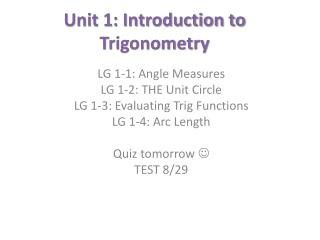# Unit 1: Introduction to Trigonometry - PowerPoint PPT PresentationDownload PresentationUnit 1: Introduction to Trigonometry

Unit 1: Introduction to TrigonometryDownload Presentation## Unit 1: Introduction to Trigonometry

- - - - - - - - - - - - - - - - - - - - - - - - - - - E N D - - - - - - - - - - - - - - - - - - - - - - - - - - -
##### Presentation Transcript

1. Unit 1: Introduction to Trigonometry LG 1-1: Angle Measures LG 1-2: THE Unit Circle LG 1-3: Evaluating Trig Functions LG 1-4: Arc Length Quiz tomorrow  TEST 8/29

2. Consider a circle, centered at the origin with 2 rays extending from the center. One ray is fixed along the positive x-axis The other can rotate about the center • These rays form an angle. The fixed ray is called the initial side of the angle. • The other side is called the terminal side. • Any angle with vertex at the origin and initial side along the positive x-axis is in standard position.

3. As the terminal side is rotated counterclockwise, the measure of the angle that is formed increases. 30o 135o 210o

4. The rotation of the terminal side may include 1 or more complete revolutions about the center. The measurement representing 1 complete revolution is 360o 1 revolution 360o 2 revolutions 720o 1 revolution + 60o 420o

5. Angles that differ by one or more complete revolutions are called coterminal angles. • For example: 74o, 434o, and 794o are all coterminal angles. Why? • Think of at least 2 coterminal angles for 105o

6. The terminal side of an angle can also rotate clockwise. A negative number is used to denote these angle measures. -45o -150o -420o

7. Suppose the angles on your cards are in standard position. Place each angle in the quadrant that contains its terminal side.

8. 90o -240o 397o -210o 800o 440o How would these angles change if they opened clockwise? First, label the points where the circle intersects the axes. 180o 0o 360o 245o -32o 198o -94o 300o 945o 275o 270o

9. How do bulldogs get flat noses? • Complete the activity in the next 10 minutes. You may work with a partner (so you don’t have to keep flipping!)

10. A unit other than degrees is also used to describe the measure of an angle. It is called the radian. Suppose there is a circle with radius of 1 centered at the origin. It’s called the Unit Circle Form an angle in standard position so that it intercepts an arc whose length is one unit. The angle made is given the measurement of 1 radian. Approximately 6.28 of these “slices” can fit all the way around the circle…

11. You will convert degrees to radians and vice versa by using this conversion:

12. Examples: • Convert the following to degrees: B. Convert the following to radians: C. Determine which quadrant each of the above angles is located.

13. Practice

14. Ticket out the Door: On a half sheet of paper, describe in words what a coterminal angle is. How do you find a coterminal angle?

15. Homework • Complete the worksheet